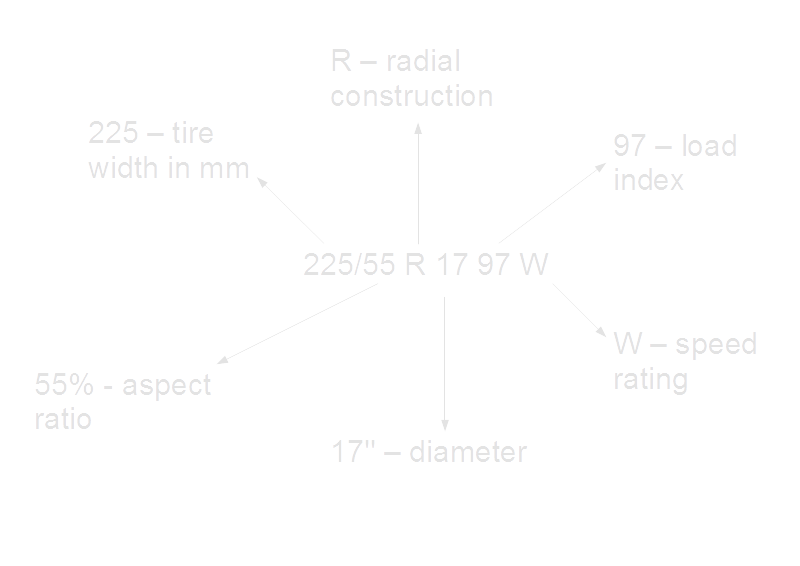Tyres speed rating table
Table summarizes how to read speed ratings codes printed on tyres and more.

# Tyres codes - speed ratings#

 Speed Symbol Approx mph Aprox km/h A1 3 5 A2 6 10 A3 9 15 A4 12 20 A5 16 25 A6 19 30 A7 22 35 A8 25 40 B 31 50 C 37 60 D 40 65 E 43 70 F 50 80 G 56 90 J 62 100 K 68 110 L 75 120 M 81 130 N 87 140 P 94 150 Q 100 160 R 106 170 S 112 180 T 118 190 H 131 210 V 149 240 VR 131 210 W 168 270 Y 186 300 ZR 149 240

# Some facts#

We explained below, how to read tire markings. The things you can read from symbol on a tire are:
• Nominal section width - this is a width of tire in milimeteres.
• Aspect ratio - this is a relation beetwen tire's high and width in percentages. Less ratio means wider tire. Historically, the most common was the profile 100% (equal width and height), but there are many different ratios popular nowadays like 80, 70, 60, 55 or 50 etc.
• Tire construction - this parameter says what is internal construction of the tire. Most common symbols are:
• R - for radial construction. It's the most popular type for cars.
• D - for diagonal construction. This type of tires is popular for example in agricultural machinery.
• B - for bias belt construction. This is special hybrid beetwen radial and diagonal construction. We can found this type of tires for example in the motorbikes.
• Diameter - for example 17'' diameter means that given tire fits a 17" diameter wheel.
• Load index - this parameter determines the maximum allowed load, which can be handled by single tire safely. It's a two or three digit symbol denoting maximum load in pounds or kilograms. For example load index 97 is related to maximum load of 730 kg (1,610 lb) per single tire. The table showing how to find maximum load in lb/kg (when we know load index) can be found in the calculator: Tyres codes - load index.
• Speed rating - this parameter determines the maximum speed, which can be applied to the tire safely with load given by load index. In most common case it's a single letter symbol. For example single letter Q means, that maximum allowed speed is 100 mph (160km/h). The table showing how to find maximum speed (when we know the tire speed rating) can be found in the calculator: Tyres codes - speed ratings.
• If you're interested in what else you can read from the imprints on your tires, check out our other calculators:

# Example#

There is example symbol, which we can found on the car tire below. We can read from it, that:
• The tire has 225 mm of width.
• Its aspect ratio (height divided by width) is 55%. So, it's rather "wide" tire.
• The tire has radial construction.
• The tire fits a 17" diameter wheel.
• The load index of this tire is 97. It means, that its maximum load is 1,610 lb (730 kg). The table showing how to convert load index value into load in kilograms or pounds can be found in the calculator: Tyres codes - load index.
• Its speed rating is W, which means that we can drive with maximum speed 168 mph (270 km/h). The table showing how to convert speed rating symbol into speed in mph or km/h can be found in the calculator: Tyres codes - speed ratings# Tags and links to this website#

Tags:
Tags to Polish version: